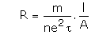# Derive ohm's law on the basis of the theory of electron drift...?

Derive ohm’s law on the basis of the theory of electron drift…?

To find the relationship between current and drift velocity, consider a conductor of length l and area of cross-section A. If V is the potential difference across the ends of the conductor, then the strength of the electric field is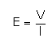The acceleration acquired by each electron due to the electric field is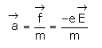whereis the coulomb’s force experienced by each electron and m is the mass of the electron.
The drift velocity of the electrons is given bywhere t, the relaxation time, is the average time that an electron spends between two collisions. It is of the order of 10-14 s.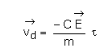Now the volume of the conductor is equal to Al and if n is the number of free electrons per unit volume, then, the total number of free electrons in the conductor will be equal to n Al.
Hence, the total charge q = - n Ale
The time taken by free electrons to cross the conductor is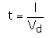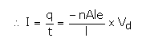where we have substituted for t and q.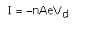For a given conductor, I a Vd
A small value of drift velocity 10-5m/sec produces a large amount of current, as there are a large number of free electrons in a conductor.
The drift velocity of the electrons Vd is (using E = V / l)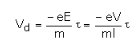Also I = - neAVd
On substituting for Vd in the above expression we get,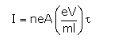or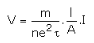Which shows that current is directly proportional to the applied voltage(Ohm’s law).From this, the resistance R can be identified as